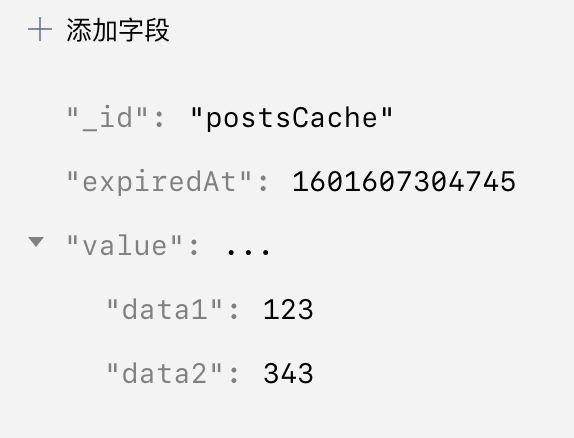# 置顶基于云开发搭建 KV 缓存系统 2.0精选热门

1. 没有进行错误处理
2. 没有对数据进行过期时间的处理
3. 如果没有数据的话，如何触发数据更新

# 对数据进行错误处理

## 设置缓存

``````async function setCache(key, value) {
try {
data: {
_id: key,
value: value
}
})
return {
code:0
}
} catch (error) {
return {
code:1,
msg: error
}
}
}
``````

## 读取缓存

``````async function getCache(key) {
try {
let { data } = await db.collection("cache").doc(key).get();
return {
code: 0,
value: data.value
};
} catch (error) {
return {
code: 1,
msg: error
}
}
}
``````

# 对数据进行过期时间的处理## 设置缓存

``````async function setCache(key, value,expire_time = 3600) {
try {
let expired_time = (new Date()) + expire_time * 1000
data: {
_id: key,
value: value,
expiredAt: expired_time
}
})
return {
code:0
}
} catch (error) {
return {
code:1,
msg: error
}
}
}
``````

## 读取缓存

``````async function getCache(key) {
try {
let { data } = await db.collection("cache").doc(key).get();
let current = (new Date()).valueOf();
if (data.expiredAt < current) {
return {
code:1,
msg: "Cache expired"
}
}
return {
code: 0,
value: data.value
};
} catch (error) {
return {
code: 1,
msg: error
}
}
}
``````

# 如何触发数据的更新

## 设置缓存

``````async function setCache(key, value,expire_time = 3600) {
try {
let expired_time = (new Date()) + expire_time * 1000
data: {
_id: key,
value: value,
expiredAt: expired_time
}
})
return {
code:0
}
} catch (error) {
return {
code:1,
msg: error
}
}
}
``````

## 获取缓存

``````async function getCache(key,uploadFunction) {
try {
let { data } = await db.collection("cache").doc(key).get();
let current = (new Date()).valueOf();
if (data.expiredAt < current) {
setCache(key,data)
return {
code:0,
value: data
}
}
return {
code:1,
msg: "Cache expired"
}
}
return {
code: 0,
value: data.value
};
} catch (error) {
return {
code: 1,
msg: error
}
}
}
``````

# 总结

1 个评论